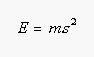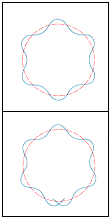Louis-Victor de Broglie

Wave Particle Duality (Louis-Victor de Broglie)

The theory of wave--particle duality developed by Louis-Victor de Broglie eventually explained why the Bohr model was successful with atoms or ions that contained one electron. It also provided a basis for understanding why this model failed for more complex systems.

De Broglie started with the fact that light acts as both a particle and a wave. In many ways light acts as a wave, with a characteristic frequency, wavelength, and amplitude. Einstein argued, however, that light carries energy as if it contains discrete photons or packets of energy. In his doctoral thesis at the Sorbonne in 1924, de Broglie looked at the consequences of assuming that light simultaneously has the properties of both a particle and a wave. He then extended this idea to other objects, such as an electron.

When an object behaves as a particle in motion, it has an energy proportional to its mass (m) and speed with which it moves through space (s).When it behaves as a wave, however, it has an energy that is proportional to its frequency.By simultaneously assuming that an object can be both a particle and a wave, de Broglie set up the following equation.

By rearranging this equation, he derived a relationship between one of the wave-like properties of matter and one of its properties as a particle.

As noted in the previous section, the product of the mass of an object times the speed with which it moves is the momentum (p) of the particle. Thus, the de Broglie equation suggests that the wavelength ( ) of any object in motion is inversely proportional to its momentum.

De Broglie concluded that most particles are too heavy to observe their wave properties. When the mass of an object is very small, however, the wave properties can be detected experimentally. De Broglie predicted that the mass of an electron was small enough to exhibit the properties of both particles and waves. In 1927 this prediction was confirmed when the diffraction of electrons was observed experimentally by C. J. Davisson.

De Broglie applied his theory of wave--particle duality to the Bohr model to explain why only certain orbits are allowed for the electron. He argued that only certain orbits allow the electron to satisfy both its particle and wave properties at the same time because only certain orbits have a circumference that is an integral multiple of the wavelength of the electron, as shown below.The fact that only certain orbits are allowed in the Bohr model of the atom can be eplained by assuming that the circumference of the orbit must be an integral (n = 1, 2, 3, 4 . . .) multiple of the wavelength of the electron.   When it is not, the electron cannot simultaneously satisfy its wave and particle behavior.